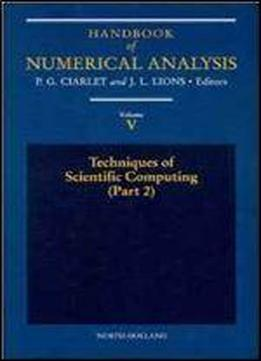# Techniques Of Scientific Computing (part 2), Volume 5 (handbook Of Numerical Analysis) (pt.2 Vol 5) by P.G. Ciarlet / 1997 / English / DjVu

This series of volumes aims to cover the major aspects of Numerical Analysis, serving as the basic reference work on the subject. Each volume concentrates on one, two, or three, particular topics. Each article, is an in-depth survey, reflecting the most recent trends in the field, and is essentially self-contained. The handbook covers the basic methods of numerical analysis, under the following general headings: solution of equations in R n finite difference methods finite element methods techniques of scientific computing and optimization theory and systems science. It also covers the numerical solution of actual problems of contemporary interest in Applied Mathematics, under the following headings:

views: 356